# Elementary divisors

(diff) ← Older revision | Latest revision (diff) | Newer revision → (diff)

of a matrix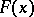over a polynomial ring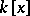Powers of the monic irreducible polynomials over the fieldinto which the invariant factors ofsplit. Two-matrices overhaving the same rank are equivalent (that is, can be obtained from one another by means of elementary operations) if and only if they have the same system of elementary divisors.

The elementary divisors of an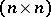-matrixoverare, by definition, those of its characteristic matrix. They can be obtained in the following manner. Letbe the greatest common divisor of the minors of orderof the matrix,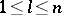, and let. Then the invariant factors of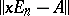areThe factorsdifferent fromare in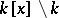. Each of them can be represented in the form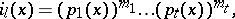where the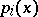are monic irreducible polynomials over,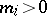, and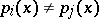when. All the polynomials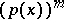thus obtained form the system of elementary divisors of. Two square matrices over a field are similar if and only if they have the same system of elementary divisors. The product of all elementary divisors of a matrix over a field is its characteristic polynomial, and their least common multiple is its minimum polynomial. Any collection of polynomials of the form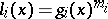, where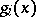is a monic irreducible polynomial over, is the system of elementary divisors for one and only one class of similar matrices overof order, whereis the degree of the product of the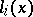.

Ifis a splitting field of the characteristic polynomial of, then the elementary divisors have the form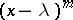. Their number is then the same as the number of Jordan cells in the Jordan form of, and the elementary divisorcorresponds to a Jordan cell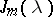of order(see Jordan matrix). A square matrix over a fieldis similar to a diagonal matrix if and only if each elementary divisor of it has the form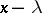, where.